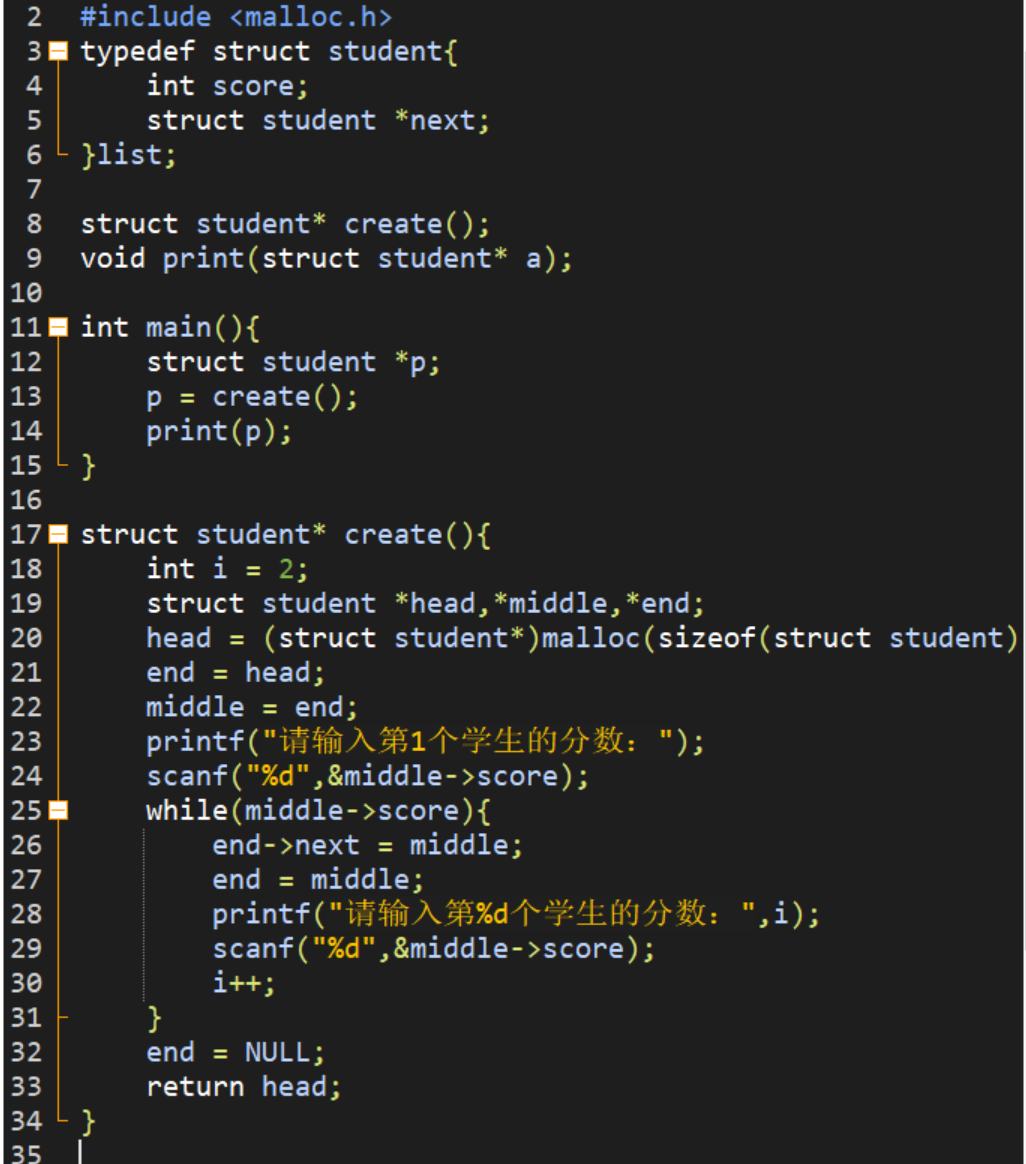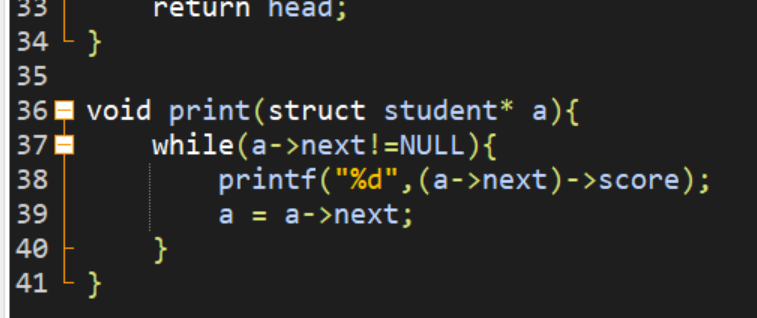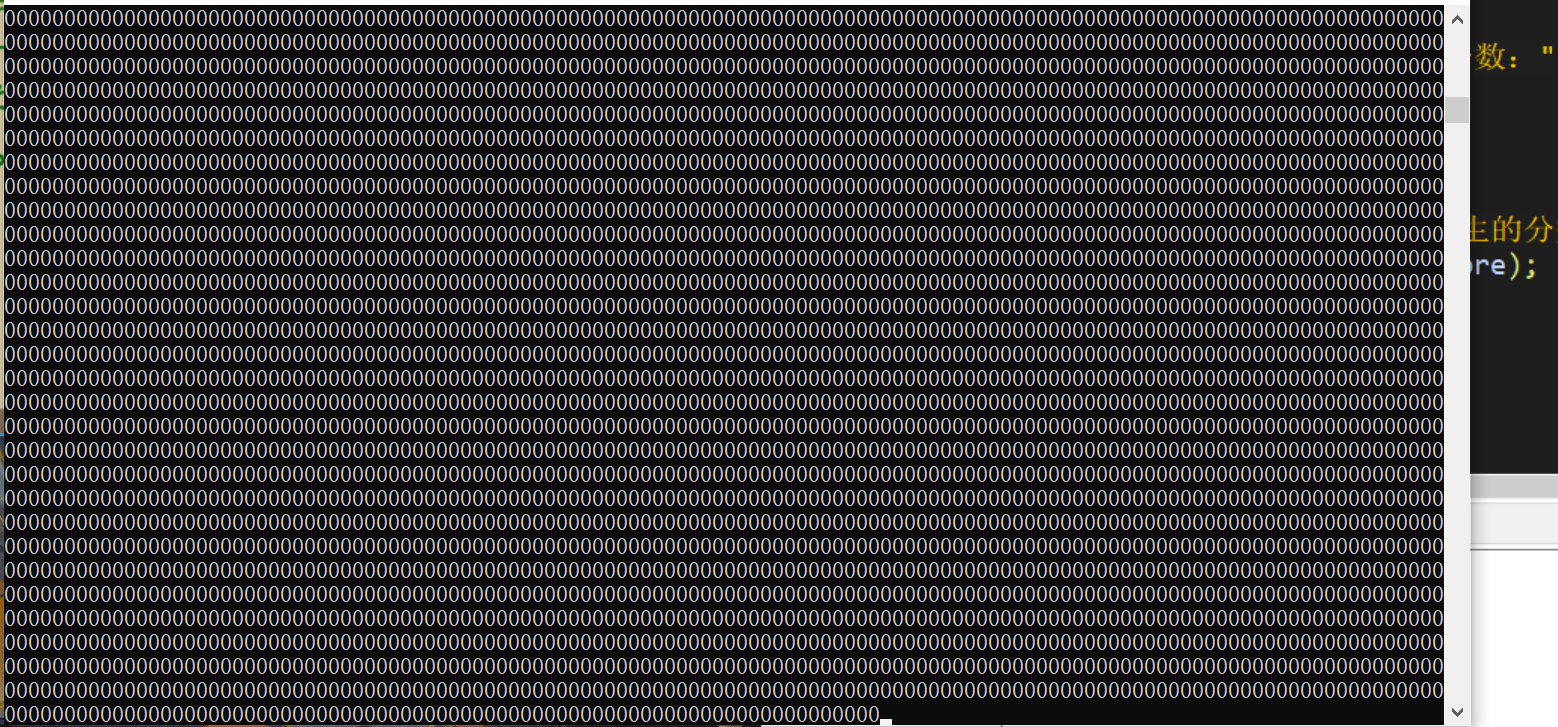2021-10-12 22:48

c语言的链表相关问题• 写回答
• 好问题 提建议
• 追加酬金
• 关注问题
• 收藏
• 邀请回答

1条回答默认 最新

•qzjhjxj 2021-10-12 23:53
已采纳

修改如下，供参考对照：

#include<stdio.h>
#include<malloc.h>
typedef struct student{
int score;
struct student *next;
}list;
struct student* create(){
int i=0;
while(1){
end = (list *)malloc(sizeof(list));
end->next = NULL;
printf("请输入第%d个学生的分数:",i+1);
scanf("%d",&end->score);
if(end->score == -1) break;   //-1 结束输入
if(i==0)
{
}
else{
middle->next = end;
}
middle = end;
i++;
}
free(end);
}
void print(struct student *a)
{
struct student *p;
p = a->next;
while(p)
{
printf("%d ",p->score);
p = p->next;
}
printf("\n");
}

int main()
{
list *p;
p = create();
print(p);

return 0;
}

评论
解决 无用
打赏 举报